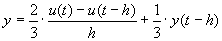﻿ 20-sim webhelp > Library > Signal > Block Diagram > differentiate-calculus

# differentiate-calculus

## Library

Signal\Block Diagram

## Use

Domains: Continuous. Size: 1-D. Kind: Block Diagrams.

## Description

This model approximates the differentiation of an input signal by the equation:where hk = simulation step size. The initial value of the output is equal to the parameter "initial". When the simulation step size is zero (time event, state event) the output value will be equal to the previous output value (the simulation step size part is not calculated to prevent a division by zero).

## Interface

 Inputs Description input Outputs output Parameters initial The initial value of the output.